# The ZX80 Operating Manual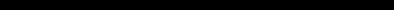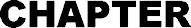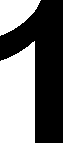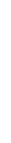A RAGBAG OF FUNCTIONS
This chapter covers all those statements and functions that haven't already been dealt with elsewhere.

 RND(X) provides a random number in the range 1 to X Typical statement using RND: 10 LET J = RND(X) sets J equal to a random number Every time the ZX-80 executes the function RND it uses a random number generator to generate a pseudo-random number. It is called pseudo-random because the numbers occur in a fixed sequence. However, the sequence is very long and hence appears random. RANDOMISE sets the starting point of the sequence to a number equal to the number of frames supplied to the TV since the machine was turned on (unless POKE is used to alter the count, see below). RANDOMISE n sets the starting point of the sequence to n unless n is 0, when it behaves as RANDOMISE. The RANDOMISE statement allows the random sequence to be initialised at any time in the program. Typical forms: 10 RANDOMISE 6 10 RANDOMISE RANDOMISE n (n ≠ o) generates a sequence depending only on the value of n (which will be the same from run to run if n is unchanged). RANDOMISE generates a different sequence each time. One highly useful statement is POKE.This is of the form POKE A,B where A is the ADDRESS of a location in store and B is an expression (the value of which should be less than 256 for sensible results). Typically A might be the address of one of the two bytes which form the variable which acts as the frame counter. This is demonstrated in the next example program.     Thus: LET X = PEEK (A) sets X equal to the contents of address A PEEK (A) is always less than 256 (in range 0 to 255) The reason that the variables associated with PEEK and POKE should always be less than 256 (255 is maximum) is that everything in the ZX-80 is stored in 8 bit bytes. Bit is short for Binary digit. The maximum which can be stored in 8 bits is 255, and so all the integer variables use up 2 bytes, which allows numbers up to 32,767 to be stored. Each half of a variable is stored in one byte, and every byte has an address of its own. Here is a program which uses PEEK and POKE to gain access to the TV frame counter. PEEK/POKE REACTION TIMER 10 FOR I = 1 to 20* RND(100) 20 NEXT I 30 POKE 16414,0 40 POKE 16415,0 50 PRINT "HIT RETURN" 60 INPUT C\$ 70 LET A= PEEK (16414) 80 LET B= PEEK (16415) 90 PRINT "YOUR REACTION TIME WAS";(B^256+A-4) *20; "MILLISECS" 16414 and 16415 are the addresses of the two halves of a 16 bit number which counts the frames on the TV  increasing by 1 every 1/50th of a second. Lines 30 to 40 set the count to zero, and the count is stopped when a null string is input to C\$. There is a delay on all operations (mainly between pressing the return key and this signal getting to the CPU) of around 60 mS, hence the 4 subtracted from the expression in line 90. This could have a repeat mechanism tacked on the end, for example: 100 PRINT "DO YOU WANT ANOTHER GO?" 110 PRINT "TYPE Y OR N" 120 INPUT C\$ 130 IF C\$ = "Y" THEN GO TO 10 140 STOP Another function which allows the user to communicate directly with the ZX-80 is USR(A) this calls a machine-code subroutine at the address A. The value is whatever the routine leaves in HL (a storage register within the central processor of the ZX-80) or, if the subroutine has not altered HL, the result is A Typical form: LET J = USR(A) PEEK, POKE and USR(A) are really facilities provided for very experienced users who understand the detailed working of the ZX-80. CLEAR is a statement which resets all the variables in the program. Typical form: 10 CLEAR (RUN automatically clears the variables every-time a program is run.) And now, last but not least, DIM. This is of the form 10 DIM A(B) sets up an array A which contains B+1 variables. Each variable is called an element of array A. Arrays can have any single-letter name and can have any number of elements (providing that there is enough room to store all the elements). It is possible, though not a good idea, to have a variable and an array with the same name example:
10 LET A = 4
20 DIM A(A)

Each element is referred to by its subscript. For instance

B(0) is the first element of array B
B(2) is the third element of array B B(N) is the (N+1)th element of array B.

Because you can use any integer expression as a subscript it is possible to process array elements easily and quickly.

Here is an example of the use of arrays for character manipulation.

CHEESE NIBBLER

10 DIM A(10)
20 DIM B(10)
30 DIM C(10)
100 FOR J = 1 T0 10
110 LET A(J)=1
120 LET B(J)=1
130 LET C(J)=1
140 NEXT J
200 FOR J=1 T0 10
205 IF NOT A(J) = 1 THEN GO TO 220
210 PRINT "";
215 GO TO 230
220 PRINT "";
230 NEXT J
240 PRINT
300 FOR J=1 T0 10
305 IF NOT B(J) = 1 THEN GO TO 320
310 PRINT "";
315 GO TO 330
320 PRINT "";
330 NEXT J
340 PRINT
400 FOR J=1 T0 10
410 PRINT "":
415 GO TO 430
420 PRINT "";
430 NEXTJ
440 PRINT
445 PRINT "HIT NEWLINE TO NIBBLE THE CHEESE"
450 INPUT Y\$
460 IF NOT Y\$="" THEN GO TO 1000
470 CLS
500 LET I = RND(10)
510 LET K=RND(3)
520 IF K = 1 THEN LET A(I) = 0
530 IF K = 2 THEN LET B(I) = 0
540 IF K = 3 THEN LET C(I) = 0
550 GO TO 200
1000 STOP

When typing in a program like this where there are several similar lines the EDIT facility is very useful, because you can edit line numbers.

For example; after entering line 210, type:

EDIT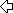RUBOUT
3
NEWLINE

Statements 10 to 30 set up 3 arrays. A, B, and C of 10 elements each. Statements 100 to 140 set all the elements of all arrays to 1.

200 to 240 examine each element of the array A in turn. If an element is equal to 1 a  is printed. If an element is not 1 a space is printed.

Similarly for 300 to 340 and 400 to 430.

On the first pass all the elements are set to 1 and  is printed all the way through.

445 to 470 print the instruction and if the user then hits NEWLINE the screen is cleared.

500 to 540 select a random element of a random array to be set to 0.

At 550 the program jumps back to print out all the arrays. The element which has been set to 0 is printed as a space - or nibble.

As more nibbles are taken there is a greater chance that the element selected will already be 0 and thus the effective nibble rate slows down as the game progresses.

ABS(n) this function gives n if n>0, and -n if n<0. n is any integer expression. e.g.

ABS(5) = 5
ABS(-5) = 5